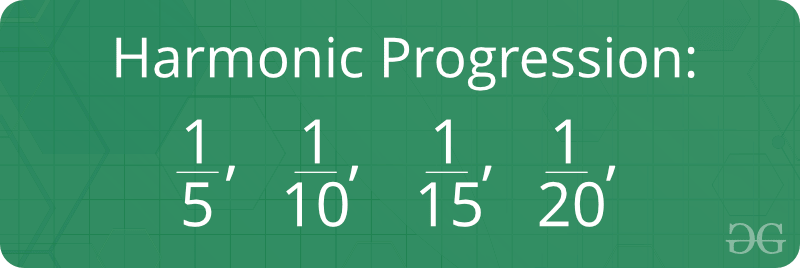Open In App

# Harmonic Progression

A sequence of numbers is called a Harmonic progression if the reciprocal of the terms are in AP. In simple terms, a, b, c, d, e, f are in HP if 1/a, 1/b, 1/c, 1/d, 1/e, 1/f are in AP. For example, 1/a, 1/(a+d), 1/(a+2d), and so on are in HP because a, a + d, a + 2d are in AP.1. In order to solve a problem on Harmonic Progression, one should make the corresponding AP series and then solve the problem.
2. As the nth term of an A.P is given by an = a + (n-1)d, So the nth term of an H.P is given by 1/ [a + (n -1) d].
3. For two numbers, if A, G and H are respectively the arithmetic, geometric and harmonic means, then
• A ≥ G ≥ H
• A H = G2, i.e., A, G, H are in GP
4. If we need to find three numbers in a H.P. then they should be assumed as 1/a–d, 1/a, 1/a+d
5. Majority of the questions of H.P. are solved by first converting them into A.P

Formula of Harmonic Progression:How we check whether a series is harmonic progression or not?
The idea is to reciprocal the given array or series. After reciprocal, check if differences between consecutive elements are same or not. If all differences are same, Arithmetic Progression is possible. So as we know if the reciprocal of the terms are in AP then given a sequence of series is in H.P. Let’s take a series 1/5, 1/10, 1/15, 1/20, 1/25 and check whether it is a harmonic progression or not. Below is the implementation:

## C++

 `// CPP program to check if a given ``// array can form harmonic progression``#include``using` `namespace` `std;` `bool` `checkIsHP(vector<``double``> &arr)``{``    ``int` `n = arr.size();` `    ``if` `(n == 1)``    ``{``        ``return` `true``;``    ``}` `    ``// Find reciprocal of arr[] ``    ``vector<``int``> rec;``    ``for` `(``int` `i = 0; i < n; i++)``    ``{``        ``rec.push_back((1 / arr[i]));``    ``}` `    ``// return (rec); ` `    ``// After finding reciprocal, check if ``    ``// the reciprocal is in A. P. ``    ``// To check for A.P., first Sort the ``    ``// reciprocal array, then check difference ``    ``// between consecutive elements ``    ``sort(rec.begin(), rec.end());``    ``int` `d = (rec) - (rec);``    ``for` `(``int` `i = 2; i < n; i++)``    ``{``        ``if` `(rec[i] - rec[i - 1] != d)``        ``{``            ``return` `false``;``        ``}``    ``}` `    ``return` `true``;``}` `// Driver Code``int` `main()``{``    ``// series to check whether it is in H.P ``    ``vector<``double``> arr = {1 / 5, 1 / 10, 1 / 15, 1 / 20, 1 / 25};` `    ``// Checking a series is in H.P or not ``    ``if` `(checkIsHP(arr))``    ``{``        ``cout << ``"Yes"` `<< std::endl;``    ``}``    ``else``    ``{``        ``cout << ``"No"` `<

## Java

 `// Java program to check if a given``// array can form harmonic progression``import` `java.util.*;` `class` `GFG``{``static` `boolean` `checkIsHP(``double` `[]arr)``{``    ``int` `n = arr.length;``    ` `    ``if` `(n == ``1``)``        ``return` `true``;` `    ``// Find reciprocal of arr[]``    ``ArrayList rec = ``new` `ArrayList();``    ``for` `(``int` `i = ``0``; i < n; i++)``        ``rec.add((``int``)(``1` `/ arr[i]));``    ` `    ``// return (rec);` `    ``// After finding reciprocal, check if``    ``// the reciprocal is in A. P.``    ``// To check for A.P., first Sort the``    ``// reciprocal array, then check difference``    ``// between consecutive elements``    ``Collections.sort(rec);``    ``int` `d = (``int``)rec.get(``1``) - (``int``)rec.get(``0``);``    ``for` `(``int` `i = ``2``; i < n; i++)``        ``if` `(rec.get(i) - rec.get(i - ``1``) != d)``            ``return` `false``;` `    ``return` `true``;``}` `// Driver code``public` `static` `void` `main(String[] args)``{``    ``// series to check whether it is in H.P``    ``double` `arr[] = { ``1``/``5``, ``1``/``10``, ``1``/``15``,``                          ``1``/``20``, ``1``/``25` `};``    ` `    ``// Checking a series is in H.P or not``    ``if` `(checkIsHP(arr))``        ``System.out.println(``"Yes"``);``    ``else``        ``System.out.println(``"No"``);``}``}` `// This code is contributed by mits`

## Python3

 `# Python3 program to check if a given``# array can form harmonic progression` `def` `checkIsHP(arr):` `    ``n ``=` `len``(arr)``    ` `    ``if` `(n ``=``=` `1``):``        ``return` `True` `    ``# Find reciprocal of arr[]``    ``rec ``=` `[]``    ``for` `i ``in` `range``(``0``, ``len``(arr)):``        ``a ``=` `1` `/` `arr[i]``        ``rec.append(a)``    ``return``(rec)` `    ``# After finding reciprocal, check if the``    ``# reciprocal is in A. P.``    ``# To check for A.P., first Sort the``    ``# reciprocal array, then check difference``    ``# between consecutive elements``    ``rec.sort()``    ``d ``=` `rec[``1``] ``-` `rec[``0``]``    ``for` `i ``in` `range``(``2``, n):``        ``if` `(rec[i] ``-` `rec[i``-``1``] !``=` `d):``            ``return` `False` `    ``return` `True` `# Driver code``if` `__name__``=``=``'__main__'``:``    ` `    ``# series to check whether it is in H.P``    ``arr ``=` `[ ``1``/``5``, ``1``/``10``, ``1``/``15``, ``1``/``20``, ``1``/``25` `]   ``    ` `    ``# Checking a series is in H.P or not``    ``if` `(checkIsHP(arr)):``        ``print``(``"Yes"``)``    ``else``:``        ``print``(``"No"``)`

## C#

 `// C# program to check if a given``// array can form harmonic progression``using` `System;``using` `System.Collections;``class` `GFG``{``static` `bool` `checkIsHP(``double``[] arr)``{``    ``int` `n = arr.Length;``    ` `    ``if` `(n == 1)``        ``return` `true``;` `    ``// Find reciprocal of arr[]``    ``ArrayList rec = ``new` `ArrayList();``    ``for` `(``int` `i = 0; i < n; i++)``        ``rec.Add((``int``)(1 / arr[i]));``    ` `    ``// return (rec);` `    ``// After finding reciprocal, check if``    ``// the reciprocal is in A. P.``    ``// To check for A.P., first Sort the``    ``// reciprocal array, then check difference``    ``// between consecutive elements``    ``rec.Sort();``    ``int` `d = (``int``)rec - (``int``)rec;``    ``for` `(``int` `i = 2; i < n; i++)``        ``if` `((``int``)rec[i] - (``int``)rec[i - 1] != d)``            ``return` `false``;` `    ``return` `true``;``}` `// Driver code``public` `static` `void` `Main()``{``    ``// series to check whether it is in H.P``    ``double``[] arr = { 1/5, 1/10, 1/15,``                        ``1/20, 1/25 };``    ` `    ``// Checking a series is in H.P or not``    ``if` `(checkIsHP(arr))``        ``Console.WriteLine(``"Yes"``);``    ``else``        ``Console.WriteLine(``"No"``);``}``}` `// This code is contributed by mits`

## PHP

 ``

## Javascript

 ``

Output:

`Yes`

Time Complexity: O(n Log n).

Auxiliary Space: O(n)
Basic Program related to Harmonic Progression